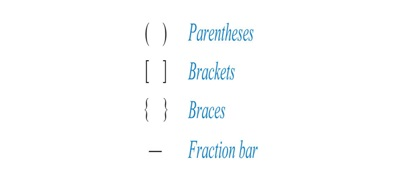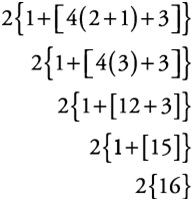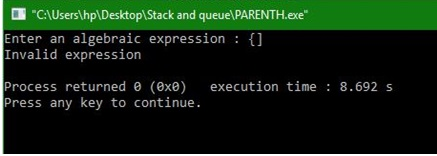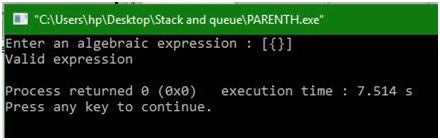# Nesting of parentheses using stack

In this article, we are going to learn how to do nesting of parentheses to check whether it is valid or invalid in data structure?
Submitted by Manu Jemini, on December 17, 2017

Parentheses are important in programming and math and sometimes the expression can get very difficult to check whether the expression is valid or not.Image source: https://web.learnbop.com/hs-fs/hub/239103/file-2521150303-png/Kate_-_Feb_2015_/operations-1.png?t=1491227798883Image source: https://2012books.lardbucket.org/books/beginning-algebra/section_04/547643d83e2356bd587467c53031527d.jpg

A simple approach to solving this type of problem is to scan the expression and store it in an array. Go to the last open parenthesis and check for the closing pair. If you find it does it for every open parenthesis. If you encountered a different type of closing parenthesis it will be invalid.

This Code implements a stack which makes the above-described approach very easy. We push all the opening and closing parenthesis into two different arrays and when we pop, we check for the similarities in type. We can also find it by checking the length of the stack.

### C program to solve nesting parenthesis using stack

```#include<stdio.h>
#include<string.h>
#define MAX 20
#define true 1
#define false 0

int top = -1;
int stack[MAX];

/*Begin of push*/
char push(char item)
{
if(top == (MAX-1))
printf("Stack Overflow\n");
else
{
top=top+1;
stack[top] = item;
}
}
/*End of push*/

/*Begin of pop*/
char pop()
{
if(top == -1)
printf("Stack Underflow\n");
else
return(stack[top--]);
}
/*End of pop*/

/*Begin of main*/
main()
{
char exp[MAX],temp;
int i,valid=true;
printf("Enter an algebraic expression : ");
gets(exp);

for(i=0;i<strlen(exp);i++)
{
if(exp[i]=='(' || exp[i]=='{' || exp[i]=='[')
push( exp[i] );
if(exp[i]==')' || exp[i]=='}' || exp[i]==']')
if(top == -1)    /* stack empty */
valid=false;
else
{
temp=pop();
if( exp[i]==')' && (temp=='{' || temp=='[') )
valid=false;
if( exp[i]=='}' && (temp=='(' || temp=='[') )
valid=false;
if( exp[i]==']' && (temp=='(' || temp=='{') )
valid=false;
}
}
if(top>=0) /*stack not empty*/
valid=false;

if( valid==true )
printf("Valid expression\n");
else
printf("Invalid expression\n");
}
/*End of main*/
```

Output 1Output 2